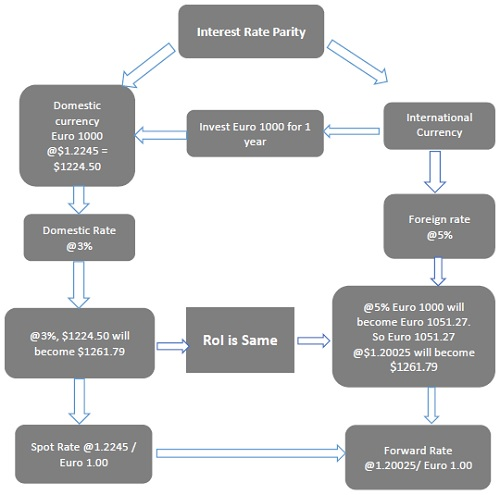# The Interest Rate Parity Model

Advertisements

## What is Interest Rate Parity?

Interest Rate Parity (IRP) is a theory in which the differential between the interest rates of two countries remains equal to the differential calculated by using the forward exchange rate and the spot exchange rate techniques. Interest rate parity connects interest, spot exchange, and foreign exchange rates. It plays a crucial role in Forex markets.

IRP theory comes handy in analyzing the relationship between the spot rate and a relevant forward (future) rate of currencies. According to this theory, there will be no arbitrage in interest rate differentials between two different currencies and the differential will be reflected in the discount or premium for the forward exchange rate on the foreign exchange.

The theory also stresses on the fact that the size of the forward premium or discount on a foreign currency is equal to the difference between the spot and forward interest rates of the countries in comparison.

### Example

Let us consider investing € 1000 for 1 year. As shown in the figure below, we'll have two options as investment cases −### Case I: Home Investment

In the US, let the spot exchange rate be \$1.2245 / €1.

So, practically, we get an exchange for our €1000 @ \$1.2245 = \$1224.50

We can invest this money \$1224.50 at the rate of 3% for 1 year which yields \$1261.79 at the end of the year.

### Case II: International Investment

We can also invest €1000 in an international market, where the rate of interest is 5.0% for 1 year.

So, €1000 @ of 5% for 1 year = €1051.27

Let the forward exchange rate be \$1.20025 / €1.

So, we buy forward 1 year in the future exchange rate at \$1.20025/€1 since we need to convert our €1000 back to the domestic currency, i.e., the U.S. Dollar.

Then, we can convert € 1051.27 @ \$1.20025 = \$1261.79

Thus, when there is no arbitrage, the Return on Investment (ROI) is equal in both cases, regardless the choice of investment method.

Arbitrage is the activity of purchasing shares or currency in one financial market and selling it at a premium (profit) in another.

## Covered Interest Rate Parity (CIRP)

According to Covered Interest Rate theory, the exchange rate forward premiums (discounts) nullify the interest rate differentials between two sovereigns. In other words, covered interest rate theory says that the difference between interest rates in two countries is nullified by the spot/forward currency premiums so that the investors could not earn an arbitrage profit.

### Example

Assume Yahoo Inc., the U.S. based multinational, has to pay the European employees in Euro in a month's time. Yahoo Inc. can do this in many ways, one of which is given below −

• Yahoo can buy Euro forward a month (30 days) to lock in the exchange rate. Then it can invest this money in dollars for 30 days after which it must convert the dollars to Euro. This is known as covering, as now Yahoo Inc. will have no exchange rate fluctuation risk.

• Yahoo can also convert the dollars to Euro now at the spot exchange rate. Then it can invest the Euro money it has obtained in a European bond (in Euro) for 1 month (which will have an equivalently loan of Euro for 30 days). Then Yahoo can pay the obligation in Euro after one month.

Under this model, if Yahoo Inc. is sure that it will earn an interest, it may convert fewer dollars to Euro today. The reason for this being the Euro’s growth via interest earned. It is also known as covering because by converting the dollars to Euro at the spot rate, Yahoo is eliminating the risk of exchange rate fluctuation.

## Uncovered Interest Rate Parity (UIP)

Uncovered Interest Rate theory says that the expected appreciation (or depreciation) of a particular currency is nullified by lower (or higher) interest.

### Example

In the given example of covered interest rate, the other method that Yahoo Inc. can implement is to invest the money in dollars and change it for Euro at the time of payment after one month.

This method is known as uncovered, as the risk of exchange rate fluctuation is imminent in such transactions.

## Covered Interest Rate and Uncovered Interest Rate

Contemporary empirical analysts confirm that the uncovered interest rate parity theory is not prevalent. However, the violations are not as huge as previously contemplated. The violations are in the currency domain rather than being time horizon dependent.

In contrast, the covered interest rate parity is an accepted theory in recent times amongst the OECD economies, mainly for short-term investments. The apparent deviations incurred in such models are actually credited to the transaction costs.

## Implications of IRP Theory

If IRP theory holds, then it can negate the possibility of arbitrage. It means that even if investors invest in domestic or foreign currency, the ROI will be the same as if the investor had originally invested in the domestic currency.

• When domestic interest rate is below foreign interest rates, the foreign currency must trade at a forward discount. This is applicable for prevention of foreign currency arbitrage.

• If a foreign currency does not have a forward discount or when the forward discount is not large enough to offset the interest rate advantage, arbitrage opportunity is available for the domestic investors. So, domestic investors can sometimes benefit from foreign investment.

• When domestic rates exceed foreign interest rates, the foreign currency must trade at a forward premium. This is again to offset prevention of domestic country arbitrage.

• When the foreign currency does not have a forward premium or when the forward premium is not large enough to nullify the domestic country advantage, an arbitrage opportunity will be available for the foreign investors. So, the foreign investors can gain profit by investing in the domestic market.

Advertisements